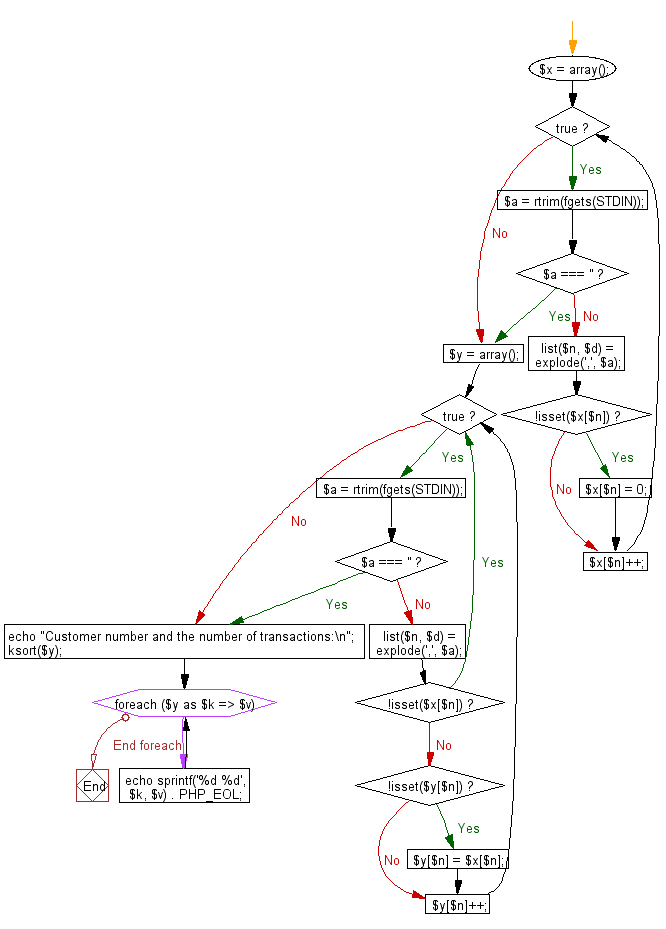﻿ PHP Exercise: Find the customer number that has traded for the second consecutive for the second consecutive month from last month and the number of transactions - w3resource

# PHP Exercises: Find the customer number that has traded for the second consecutive for the second consecutive month from last month and the number of transactions

## PHP: Exercise-70 with Solution

Record section of ABC company records the customer number and the trading date for each month. Write a PHP program to find the customer number that has traded for the second consecutive for the second consecutive month from last month and the number of transactions.
The data of this month and the data of last month are separated by a blank line of one line and given.
Transaction format:
c1 , d1
c2 , d2
...
...
ci (1 ≤ ci ≤ 1,000) is an integer represents the customer number, di (1 ≤ di ≤ 31) is an integer represents the trading date.

Sample Solution: -

PHP Code:

``````<?php
\$x = array();
while (true) {
\$a = rtrim(fgets(STDIN));
if (\$a === '') {
break;
}
list(\$n, \$d) = explode(',', \$a);
if (!isset(\$x[\$n])) {
\$x[\$n] = 0;
}
\$x[\$n]++;
}

\$y = array();
while (true) {
\$a = rtrim(fgets(STDIN));
if (\$a === '') {
break;
}
list(\$n, \$d) = explode(',', \$a);
if (!isset(\$x[\$n])) {
continue;
}
if (!isset(\$y[\$n])) {
\$y[\$n] = \$x[\$n];
}
\$y[\$n]++;
}
echo "Customer number and the number of transactions:\n";
ksort(\$y);
foreach (\$y as \$k => \$v) {
echo sprintf('%d %d', \$k, \$v) . PHP_EOL;
}

?>
```
```

Sample Input:
125,10
55,12
34,14

125,3
55,4
125,5

Sample Output:

```Customer number and the number of transactions:
55 2
125 3```

Flowchart:PHP Code Editor:

Have another way to solve this solution? Contribute your code (and comments) through Disqus.

What is the difficulty level of this exercise?

Test your Programming skills with w3resource's quiz.

﻿

## PHP: Tips of the Day

Returns all elements in an array except for the first one

Example:

```<?php
function tips_tail(\$items)
{
return count(\$items) > 1 ? array_slice(\$items, 1) : \$items;
}

print_r(tips_tail([1, 5, 7]));
?>
```

Output:

```Array
(
 => 5
 => 7
)
```